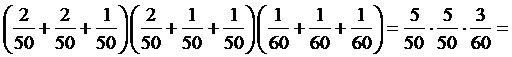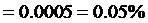3-reel slot machines

2. Case B – different numbers of stops and symbol distributions on the reels

2.6  Event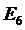– Any combination of two specific symbols

The probability ofis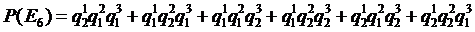, whereare the basic probabilities of the two symbols occurring on the reels respectively (is the probability of symbol no. i occurring on reel no. j).

Example:

Find the probability of any combination of red seven and blue seven symbols occurring on a payline of a 3-reel slot machine having 56, 56, and 60 stops on reels 1, 2, and 3 respectively and the following distributions of the two symbols on these reels respectively: 2, 2, 2 for red seven; 2, 1, 1 for blue seven.

The basic probabilities are: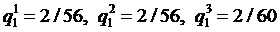and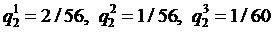. The sought probability is: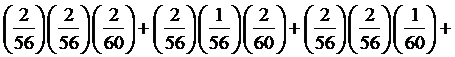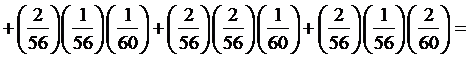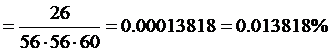.

2.7  Event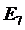– Any combination of at least one of three specific symbols

The probability ofis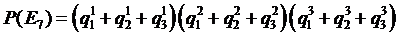, whereare the basic probabilities of the three symbols occurring on each reel respectively. Denoting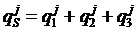, for j from 1 to 3, we can write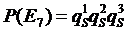and use the tables and the results of section 2.1.

Example:

Find the probability of any combination of bar-type symbols (single, double, and triple bar) occurring on a payline of a 3-reel slot machine with 50, 50, and 60 stops on reels 1, 2, and 3 respectively, and the following distributions of the bar-type symbols on these reels respectively: 2, 2, 1 for single bar; 2, 1, 1 for double bar; 1, 1, 1 for triple bar.

We have: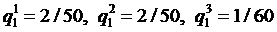,,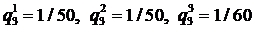The sought probability is: